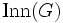# Nilpotent automorphism group implies nilpotent of class at most one more

This article gives the statement and possibly, proof, of an implication relation between two group properties. That is, it states that every group satisfying the first group property (i.e., group whose automorphism group is nilpotent) must also satisfy the second group property (i.e., nilpotent group)
View all group property implications | View all group property non-implications
Get more facts about group whose automorphism group is nilpotent|Get more facts about nilpotent group
This article gives a result about how information about the structure of the automorphism group of a group (abstractly, or in action) can control the structure of the group
View other such results

## Statement

Suppose$G$ is a group whose automorphism group is nilpotent, i.e., it has a nilpotent automorphism group. Then,$G$ is itself a Nilpotent group (?). Moreover, the Nilpotency class (?) of$G$ is at most one more than the nilpotency class of$\operatorname{Aut}(G)$.

## Facts used

1. Group acts as automorphisms by conjugation
2. Nilpotency is subgroup-closed (and the class of the subgroup is at most the class of the whole group).

## Proof

Given: A group$G$ whose automorphism group is nilpotent of class$c$.

To prove:$G$ is nilpotent of class at most$c + 1$.

Proof: We need to show that$G$ is nilpotent and the upper central series of$G$ has length at most$c + 1$. To do this, it suffices to show that the upper central series of the quotient$G/Z(G)$ (where$Z(G)$ is the center of$G$) has length at most$c$.

By fact (1), there is a homomorphism$G \to \operatorname{Aut}(G)$ given by conjugation action. The kernel of this homomorphism is$Z(G)$ and the image is a subgroup of$\operatorname{Aut}(G)$ known as the inner automorphism group, and denoted$\operatorname{Inn}(G)$. Thus,$G/Z(G)$ is isomorphic to the subgroup$\operatorname{Inn}(G)$ of$\operatorname{Aut}(G)$. By fact (2),$\operatorname{Inn}(G)$, and hence$G/Z(G)$, is nilpotent of class at most$c$, completing the proof.